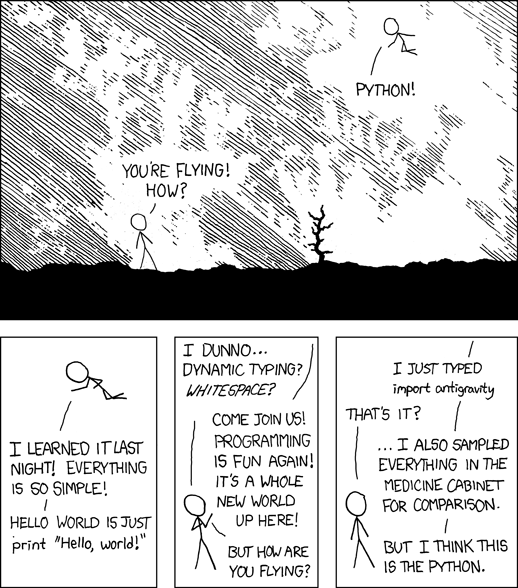Page 1 of 1

### Generate WAVE files in pure Python

Posted: Fri Mar 21, 2008 2:22 pm
Not that it's very difficult using the standard library wave and array modules. So for future reference when you want to experiment with sound generation (like synthesizing IRs):

Code: Select all

``````"""Output an empty 1 second 16-bit WAVE file."""

import wave
import array

seconds = 1.0
samples_per_second = 44100
number_of_samples = int(samples_per_second * seconds)

sample_array = array.array('h')
for n in xrange(number_of_samples):
sample_array.append(0)

wave_out = wave.open("outfile.wav", "w")
wave_out.setnchannels(1)
wave_out.setsampwidth(2) # 2 bytes for -32768 to 32767 amplitude
wave_out.setframerate(samples_per_second)
wave_out.setcomptype("NONE", "Uncompressed")
wave_out.writeframes(sample_array.tostring())
wave_out.close()``````
And if you are using SciPy (which you should) it's even simpler to write an array:

Code: Select all

``````from scipy.io import wavfile
wavfile.write("outfile.wav", samples_per_second, sample_array)``````
Finaly, an illustration: